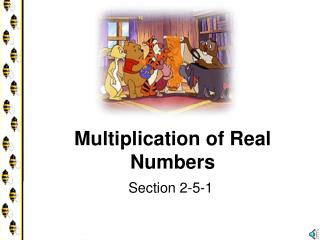DownloadDownload PresentationMultiplication of Real Numbers

# Multiplication of Real Numbers

Download Presentation## Multiplication of Real Numbers

- - - - - - - - - - - - - - - - - - - - - - - - - - - E N D - - - - - - - - - - - - - - - - - - - - - - - - - - -
##### Presentation Transcript

1. Multiplication of Real Numbers Section 2-5-1

2. Multiplication • When you first learned multiplication, your book had pictures of equal number of objects in several rows. • You learned that 3  4 meant “three fours.” • 3  4 = 4+4+4

3. Negative Numbers • 3  (-4) means “three negative fours” • 3  (-4) = (-4)+(-4)+(-4) = -12 • using the addition rules.

4. Think: Is Division Commutative?

5. Three Ways • There are three ways to write multiplication. 3x4 or 3(4) or 34

6. Commutative • Remember: multiplication is COMMUTATIVE. • 34 =43 and 3 (-4)=-43

7. Practice: • Perform the indicated operation. • 46= • 4(-6) • -6x4 • 243100 • 243(-100) • -100243

8. Negative Times Negative • So far you have learned • positive times positive, • positive times negative, • negative times positive. • There is only one pattern left. • Negative times a Negative is Positive

9. Negative Times Negative • A little hard for students to envision • Think of pouring juice out of a clear jug. • This would be negative. • Now video tape the jug emptying. • Playing the tape backwards is also a negative • the jug appears to be filling (positive) when watched on the screen. • We will discuss in more detail why this is in section 2.7

10. Rule: • a POSITIVE times a POSITIVE is POSITIVE • a NEGATIVE times a NEGATIVE is POSITIVE • a POSITIVE times a NEGATIVE is NEGATIVE • a NEGATIVE times a POSITIVE is NEGATIVE

11. Division Rules • The multiplication and division rules are exactly the same.

12. -2(-100) = 200 -5(-5) = 25 -23(-12) = 276 -3x 4 = -12 0.3 x (-2)=-0.6 -8(-4) = 2 -124 = -3 8(-4) = -2 Examples: